## Tables index

#### Norman N. Haidous, Shlomo S. Sawilowsky

American Journal of Applied Mathematics and Statistics. 2013, 1(5), 99-102 doi:10.12691/ajams-1-5-4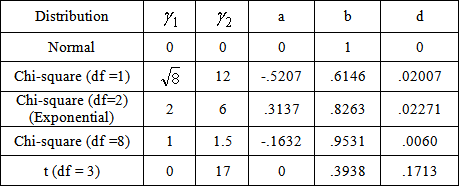• Table 1. Solutions To The Fleishman Equation For Selected Distributions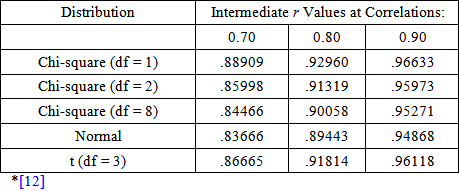• Table 2. Intermediate r Values for Various Distributions at Correlations 0.70, 0.80, and 0.90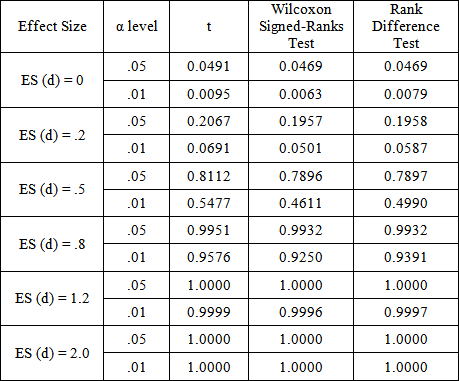• Table 3. Robustness and Power for (n1 = n2) = (15,15), Normal, rxy = .8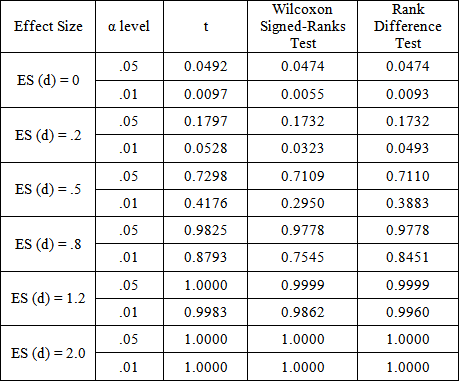• Table 4. Robustness and Power for (n1 = n2) = (10,10), Chi-squared df = 1, rxy = .7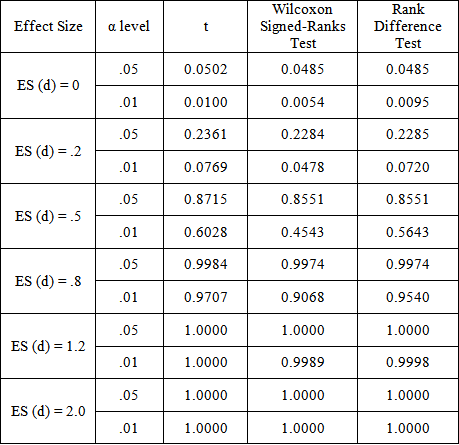• Table 5. Robustness and Power for (n1 = n2), Chi-squared df = 2, rxy = .8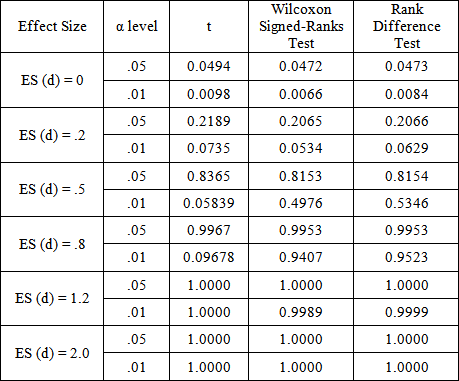• Table 6. Robustness and Power for (n1 = n2)= (15,15), Chi-squared df = 8, rxy = .7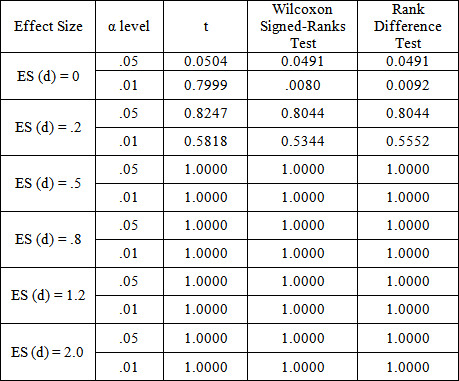• Table 7. Robustness and Power for (n1 = n2)= (20,20), t (df = 3), rxy = .7